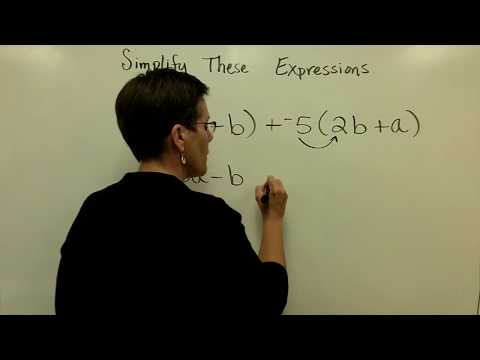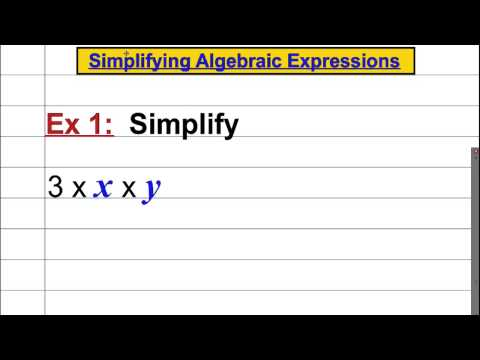## Algebraic Expressions

Subject: Compulsory Maths

#### Overview

Algebraic expressions are the symbol or a combination of symbols used in algebra containing one or more numbers, variables, and arithmetic operations. While performing the operations of multiplication and division of algebraic expression it needs to work out indices of the same bases under the certain rules. These are called the law of indices. When the coefficients of the terms of the terms are multiplied and the power of the same bases are added then it is called Multiplication of algebraic expressions. While dividing a monomial by another monomial, divide the coefficient of divided by the coefficient of divisor. Then substract the power of the base of divisor from the power of the same base of dividend.

#### Algebraic Expression

Generally, Algebraic expressions are the symbol or a combination of symbols used in algebra containing one or more numbers, variables, and arithmetic operations. 2xyz, 2x +yz, 2x + y, etc. are the examples of Algebraic Expressions.

Evaluation of Algebraic Expressions

When we replace the variable of a term or expression with numbers, the value of the terms or expression is obtained. It is called the evaluation of a term or expression. For examples,

If x = 3, y = 4 and z = 2, then

2xyz = 2 × 3 × 4 × 2 = 48

Law of Indices

While performing the operations of multiplication and division of algebraic expression it needs to work out indices of the same bases under the certain rules. These are called the law of indices.1. Product law of indices
In product law of indices, when the same base is multiplied we should add their indices. When am and an are two terms then aa + n. For examples,
2 × 2 = 21 + 1 = 22
2×2 = 24+2 = 26
2. Quotient law of indices
In quotient law of indices, when the base is divided by another same base, power should be subtracted. Like, am ÷ an = am-n. For examples,
32÷3 = $\frac{3^2}{3}$ =$\frac{3×3}{3}$ = 3 = 32-1
3. Power law of indices
In power law of indices, when a base with some power has another power, the powers are multiplied. For examples,
(22)2 = 22 × 22= 22+2= 24
4. Law of Zero indices
In this law of indices, the value of a base with power 0 is always1. If a0 is any term with a base a and power 0, then a0 = 1. For example,
20 = 1, 50 = 1

Addition and Subtraction of algebraic expression

To add and subtract the algebraic expression, there should be the like and unlike terms. Unlike terms are those which do not have the same base and like terms are those which have the same base. While adding and subtracting algebraic term, we should add or subtract the coefficients of

like terms. For example,

1. 2x + 3x = 5x
2. 7x - 5x = 2x

Multiplication of algebraic expressions

When the coefficients of the terms of the terms are multiplied and the power of the same bases are added then it is called Multiplication of algebraic expressions. For example,

1. 2x2×3x = 6x3
2. 3a2×3a2 = 9a4

Multiplication of polynomials by monomials

In this case, in each term of polynomials is separately multiplied by the monomial. For example

Multiply, (b+c) by x.

Here, x× (b+c) = bx +cx

Multiplication of polynomials

In this case, each term of polynomials is separately multiplied by each term of another polynomial. Then, the product is simplified. For examples,

(a+b) by (x+y)

Here, (x+y)(a+b)

or, x(a+b) = y(a+b)

or, ax+bx+ay+by

Some special products formulae

1. The product of (a+b) and (a+b) (square of binomials).
Let's multiply (a+b) by (a+b)
(a+b)×(a+b) = a(a+b) + b(a+b)
(a+b)2 = a2 + 2ab + b2
= a2 + 2ab + b2
Thus, (a+b)2 = a2 +2ab +b2, then
a2 + b2 = (a+b)2 - 2ab
2. The product of (a-b) and (a-b)
Let's multiply (a-b) by (a-b)
(a-b)×(a-b) = a(a-b) - b(a-b)
(a-b)2 = a2 - ab - ab + b2
= a2 - 2ab + b2
THus, (a-b)2 = a2 - 2ab + b2
Here, if (a-b)2 = a2 - 2ab + b2, then,
a2 + b2 = (a-b)2 + 2ab
3. The product of (a+b), (a+b) and (a+b) (cube of binomials)
Lets, find the products of (a+b)3
(a+b)3 = (a+b) (a+b) (a+b)
(a+b) (a+b)2
(a+b)(a2+2ab+b2)
a(a2+2ab+b2) + b(a2+2ab+b2)
a3 + 2a2b +ab2 + a2b + 2ab2 +b3
a3 +3a2b + 3ab2 + b3
∴(a+b)3 = (a3 +3a2b + 3ab2 + b3)
4. The product of (a-b), (a-b) and (a-b)
Lets, find the products of (a-b)3
(a-b)3 = (a-b) (a-b) (a-b)
(a-b) (a-b)2
(a-b)(a2-2ab+b2)
a(a2-2ab+b2) - b(a2-2ab+b2)
a3- 3a2b +ab2 + a2b + 2ab2- b3
a3- 3a2b + 3ab2- b3
∴(a-b)3 = (a3-3a2b + 3ab2- b3)
We can express these formulaes in other following forms
we have (a3 +3a2b + 3ab2 + b3) =(a+b)3
a3 + b3 + 3ab (a+b) =(a+b)3
a3 + b3 =(a+b)3 - 3ab (a+b)
∴ a3 + b3 =(a+b)3- 3ab (a+b)
Also,(a3-3a2b + 3ab2- b3) = (a-b)3
a3 - b3 - 3ab(a-b) = (a-b)3
a3 - b3 - 3ab(a-b) = (a-b)3 + 3ab(a-b)
∴ a3- b3 =(a-b)3+ 3ab (a-b)
5. The product of (a+b) and (a+b)
Let's multiply (a-b) by (a-b)
(a+b)(a-b) = a(a-b) + b(a-b) = a2 - ab + ab - b2 = a2 - b2
Thus, (a+b) (a-b) = a2 -b2

Division of algebraic expressions

While dividing a monomial by another monomial, divide the coefficient of dividend by the coefficient of of divisor. Then substract the power of the base of divisor from the power of the same base of dividend. For examples,

18x4y3 by 6x2y2

or, 18x4y3÷ 6x2y2 = $\frac{18x^4y^3}{6x^2y^2}$

or, 3x4-2y3-2 = 3x2y

Division of polynomials by monomials

In this case each term of a polynomial is separately dividend by the monomial. For example,

(12x4 - 15x3)÷ 3x2

= $\frac{24x^4}{3x^2}$ - $\frac{15x^3}{3x^2}$

= 4x4-2 - 5x3-2

= 4x2 - 5x

Division of polynomials by polynomials

In this case, at first we should arrange the terms of divisor and dividend in descending or ascending order of power of common bases. Then we should atart the division dividing the term of dividend with the highest power.

##### Things to remember
• (a+b)2 = a2 + 2ab + b2
• a2 + b2 = (a+b)2 - 2ab
• (a-b)2 = a2 - 2ab + b2
• a2 + b2 = (a-b)2 + 2ab
• (a+b)3 = (a3 +3a2b + 3ab2 + b3)
• a3 - b3 = (a-b)3 + 3ab (a-b)
• (a+b) (a-b) = a2 -b2
• It includes every relationship which established among the people.
• There can be more than one community in a society. Community smaller than society.
• It is a network of social relationships which cannot see or touched.
• common interests and common objectives are not necessary for society.
##### Videos for Algebraic Expressions##### 13 - Introductory Algebra - Simplify Algebraic Expressions##### Simplifying Algebraic Expressions
 Divisor Dividend 2 1296 2 648 2 324 2 162 3 81 3 27 3 9 3

$\therefore$ 1296 = 2×2×2×2×3×3×3×3

= 24×34

=(2×3)4

= 6 ans.

Solution:

($\frac{16}{81}$)$\frac{1}{4}$

= ($\frac{2^4}{3^4}$)$\frac{1}{4}$

= ($\frac{2}{3}$)4×$\frac{1}{4}$

= ($\frac{2}{3}$)1

= $\frac{2}{3}$

Solution:

Here,

3x2y + 5x2y + 7xy2 + (-2xy2)

= 8x2y + 7xy2 -2xy2.

= 8x2y + 5xy2

Solution:

= 7x2 - 2x + 4(3x2 + 5x - 7)

= 7x2 - 2x + 4 - 3x2 - 5x - 7

= 4x2 - 7x - 3   ans.

Solution:

= (4x - 7) (2x2 + 3x - 5)

= 4x(2x2 + 3x - 5) - 7 (2x2 + 3x - 5)

= 8x3 + 12x2 - 20x - 14x2 - 21x + 35

= 8x3 - 2x2 - 41x + 35

Solution:

= (x+y)2 - 2(x+y)z + z2

= x2 + 2xy + y2 - 2xz - 2yz + z2

= x2 + y2 + z2 + 2xy - 2yz - 2zx

Solution:

Here, (a+b) = 2

$\therefore$ (a+b)3 = 23

or, a3 + 3a2b + 3ab2 + b3 = 8

or, a3 + b3 + 3ab2 = 8

or, a3 + b3 + 6ab = 8

So, the required value of a3 + b3 + 6ab is 8.

Solution:

= $\frac{12x^4}{3x^2}$ - $\frac{15x^3}{3x^2}$

= 4x4-2 - 5x3-2

= 4x2 - 5x